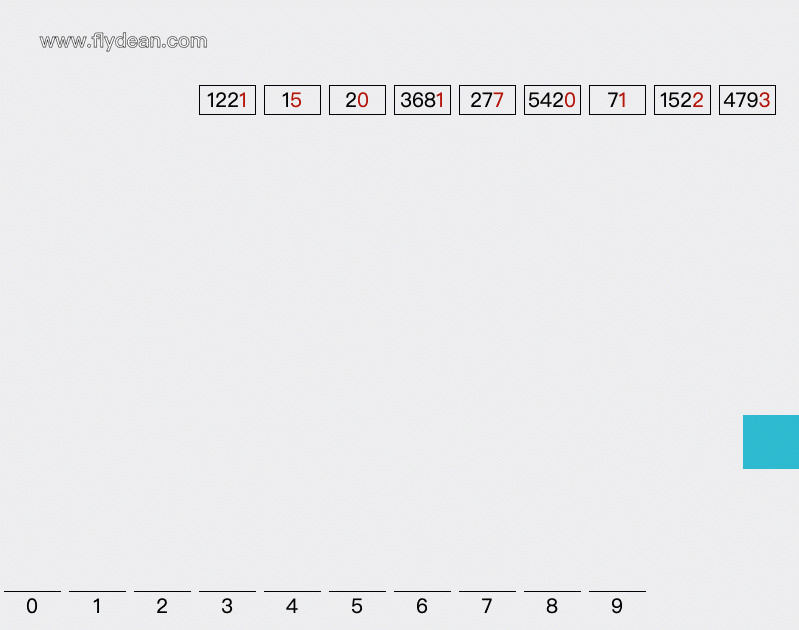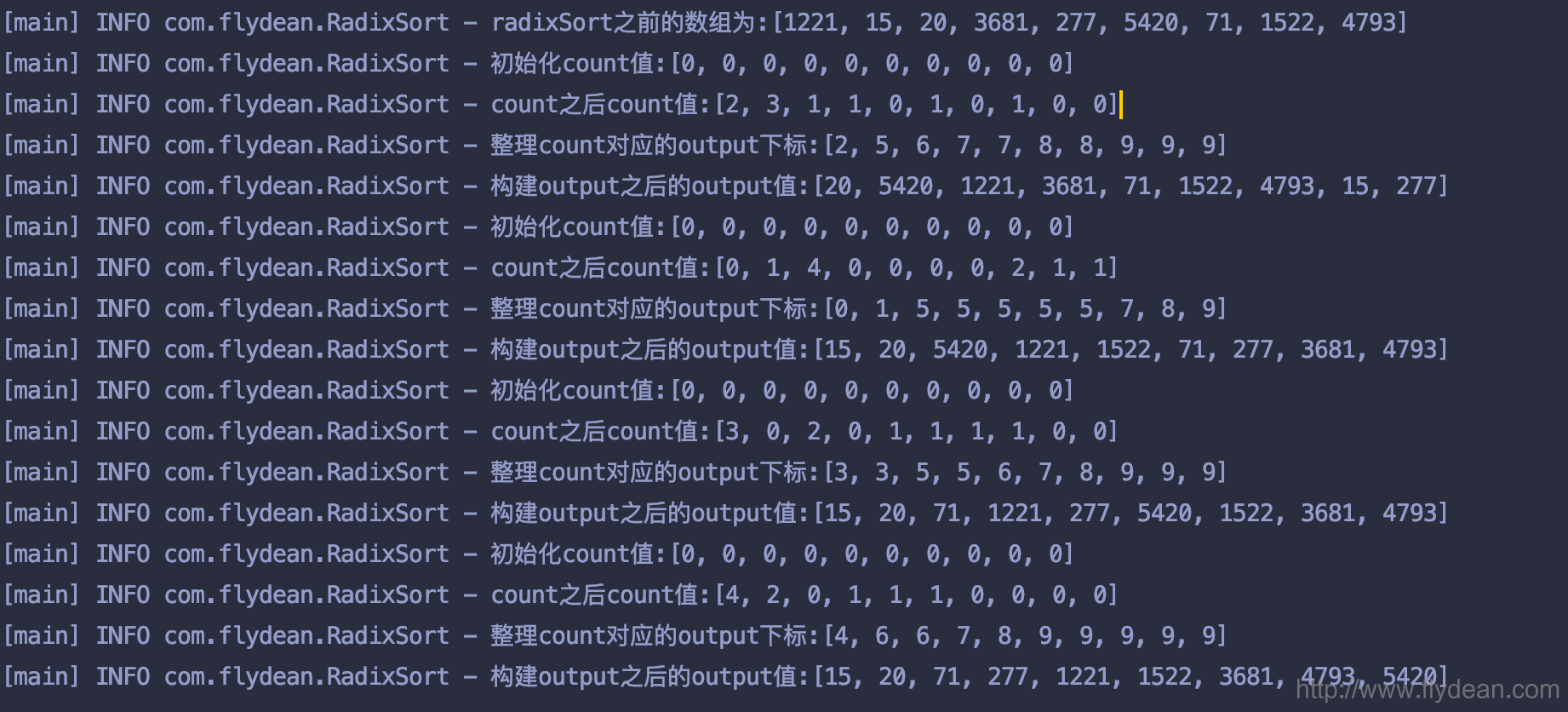# 看动画学算法之: 排序 - 基数排序# 基数排序的例子# 基数排序的 java 代码实现

doCountingSort 方法除了传入数组外，还需要传入排序的位数 digit，我们用 1，10，100，1000 来表示。

   public void doRadixSort(int[] array, int digit){        int n = array.length;        // 存储排序过后的数组        int output[] = new int[n];        // count数组，用来存储统计各个元素出现的次数        int count[] = new int;        Arrays.fill(count,0);        log.info("初始化count值:{}",count);        // 将原始数组中数据出现次数存入count数组        for (int i=0; i<n; ++i) {            count[(array[i]/digit)%10]++;        }        log.info("count之后count值:{}",count);        // 这里是一个小技巧，我们根据count中元素出现的次数计算对应元素第一次应该出现在output中的下标。        //这里的下标是从右往左数的        for (int i=1; i<10; i++) {            count[i] += count[i - 1];        }        log.info("整理count对应的output下标:{}",count);        // 根据count中的下标，构建排序后的数组        //插入一个之后，相应的count下标要减一        for (int i = n-1; i>=0; i--)        {            output[count[(array[i]/digit)%10]-1] = array[i];            count[(array[i]/digit)%10]--;        }        log.info("构建output之后的output值:{}",output);        //将排序后的数组写回原数组        for (int i = 0; i<n; ++i)            array[i] = output[i];    }

public int getMax(int[] array)    {        int mx = array;        for (int i = 1; i < array.length; i++)            if (array[i] > mx){                mx = array[i];            }        return mx;    }

    public static void main(String[] args) {        int[] array= {1221, 15, 20, 3681, 277, 5420, 71, 1522, 4793};        RadixSort radixSort=new RadixSort();        log.info("radixSort之前的数组为:{}",array);        //拿到数组的最大值，用于计算digit        int max = radixSort.getMax(array);        //根据位数，遍历进行count排序        for (int digit = 1; max/digit > 0; digit *= 10){            radixSort.doRadixSort(array,digit);        }    }# 基数排序的时间复杂度

d 是需要循环的轮数，也就是数组中最大数的位数。假如数组中最大的数字用 K 表示，那么 d=logb(k)。## 评论